# PIC32MX: Sinusoidal Analog Output

The PIC32 is actually incapable of directly creating an analog output. Instead, the PIC32 can perform pulse width modulation (PWM) to create an average analog output. At a given frequency, PWM varies the time that the output pin is on (output is 3.3V) to create the duty cycle which can simulate voltages between 0V and 3.3V. For example, if the duty cycle is 50%, the PIC32 holds the pin at 3.3V 50% of the time and 0V for the other 50%. The average output voltage is then 1.65V. If the pin is connected to an external RC circuit, the PWM can be integrated. By varying the duty cycle sinusoidally, the output with an RC circuit can resemble a sinusoid. Thus, to create the sinusoidal output, a sinusoidal wave must be divided into a discrete number of points. These points can then be converted to a list of duty cycles to represent the sinusoid.

The three most important parameters in creating the sinusoidal analog output are the the number of points per period, the RC time constant, and the sinusoid frequency. The combination of the sinusoid frequency and the points per period helps tell the PIC when to change the duty cycle. The RC constant of an external RC circuit is important in creating smooth and accurate curves and in improving the quality of the output signal.

The code for the analog output will first generate a lookup table. This table contains values for the specific duty cycles and will generate the number of duty cycles based on the number of points specified by the user. After generating the table, the code goes through a loop which tells the board to output the appropriate PWM corresponding to the duty cycle in the lookup table at each time step. The timing for the loop is determined by an interrupt which runs a frequency, $f$, which tells the PIC when to switch to the next appropriate PWM from the lookup table. By looping through the duty cycles at the same time intervals, the output voltage is varied to match that of a sine wave. The external RC circuit acts to store the charges from the PWM and smooth the final output voltage to yield the sine wave. Without this external RC circuit, the output would only be a series of pulses matching the duty cycle at a given time.

The equation governing the sine wave is $y=A*sin({\frac {2\pi }{N*i}})$where $A$is the amplitude of the sinusoid, $N$is the number of points and $i$is the index.

The equation governing the duty cycle is $dutycycle={\frac {MAX\_DUTY}{2}}y+{\frac {MAX\_DUTY}{2}}$where the $MAX\_DUTY$is determined by the frequency of the PWM.

The examples below have a PWM frequency of 20kHz.

## Number of Points Per Period

Selecting the number of points to use to generate the sine wave is important because choosing too few will result in a very rough sine wave. When the number of points is too low, you can actually see multiple RC charging graphs between each point. However, if we choose too many points, a peak appears at what should be 0V on the sine wave. To prevent this peek from occurring, one could insert an “if” statement in the lookup table generation loop that replaces all of the occurring 0’s into 1’s. Inclusion of the if statement would result in no true 0V output for that particular output. The ideal number of points for 25Hz is around 200 points.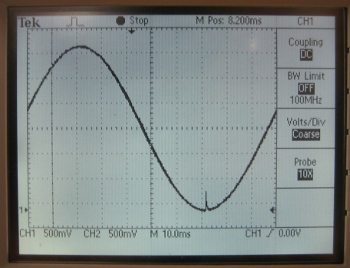This demonstrates what happens when you pick too many points. The output signal has a peak occurring at the 0V area. This output signal used 500 points at 10Hz.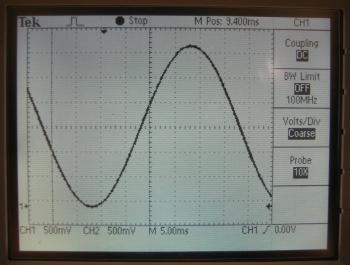This demonstrates what an ideal analog output should look like. This output was generated using 100 points at 25Hz.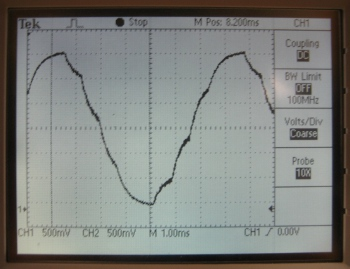This demonstrates what happens when you select too few points. The output signal shows the capacitor charging. In this case, 10 points at 25Hz are used in generating the analog output.

## RC Time Constant

The RC time constant plays a big role in creating the sine wave. By increasing the RC constant, the quality of the output signal is increased, where "quality" refers to the oscillations that appear around the sine wave that occur because of PWM. A larger oscillation around the sine wave means that it is of poorer quality. Despite having such advantages, a larger RC constant will result in a phase lag which causes a delay between the output of the PIC and the output from the whole circuit.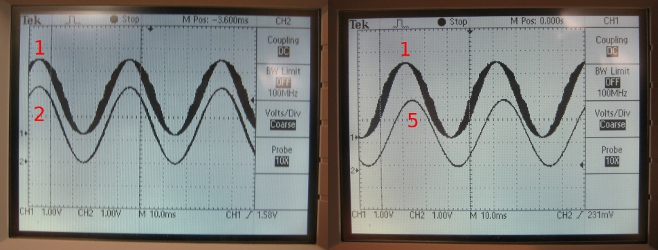The sine waves were generated at 25Hz with 100 points. The sine wave labeled 1 was generated with an RC constant of 4.7 x 10^-5, the sine wave labeled 2 was generated using an RC constant 2.2 x 10^-4 and the sine wave labeled 5 was generated using an RC constant 4.7 x 10^-3. Notice that sine waves 1 and 2 are almost synchronized, but sine waves 1 and 5 have a phase lag caused by the high RC constant. The improvement in quality is also visible in these two graphs. Sine wave 5 has an RC constant that's 100 times larger than that of sine wave 1, and there's very significant improvement in the quality of the output signal.

## Frequency

There are two important frequencies that affect the performance of the PIC in generating the analog output. The first is the cut-off frequency, and the second is the sine frequency.

The cut-off frequency is a function of the RC time constant. The equation to determine the cut-off frequency is

$f_{c}={\frac {1}{2\pi RC}}{\text{ Hz}}$where $f_{c}$is the cut-off frequency (${\text{Hz}}$), $R$is the resistance ($\Omega$'s) and $C$is the capacitance (F).

This cut-off frequency can cause a loss in the output amplitude. The determination of if the cut-off frequency is too high depends on the sine frequency. If the cut-off frequency is too high (RC constant is too low) relative to the sine frequency, then the output signal may be very noisy and may also have a decreased amplitude. If the cut-off frequency is too low (RC constant is too high) relative to the sine frequency, then the final output signal through the RC circuit will be more likely to have a decreased amplitude, thus you'll only get a limited range of voltages. Below are some results from experimentation with varying sine frequencies.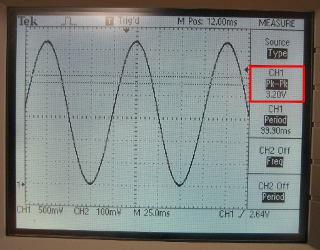Oscilloscope reading of the sinusoidal analog output with a Sine Frequency of 10Hz with a resistor of 47 kOhms and a capacitor of 0.1 microFarads. The Peak to Peak Voltage reading is 3.2V.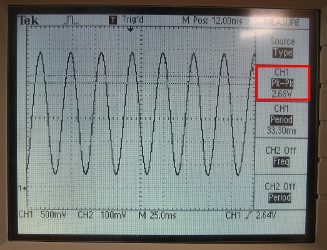Oscilloscope reading of the sinusoidal analog output with a Sine Frequency of 30Hz with a resistor of 47 kOhms and a capacitor of 0.1 microFarads. The Peak to Peak Voltage reading is 2.68V.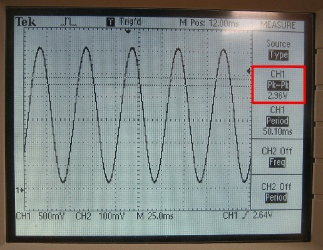Oscilloscope reading of the sinusoidal analog output with a Sine Frequency of 20Hz with a resistor of 47 kOhms and a capacitor of 0.1 microFarads. The Peak to Peak Voltage reading is 2.96V.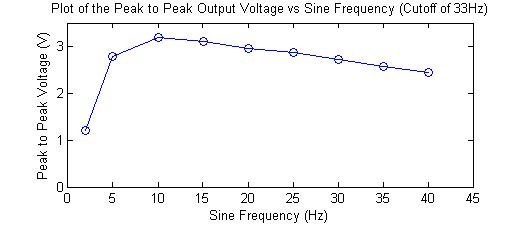Graph of the results from an experiment. This experiment had a cutoff frequency of 33Hz (47kOhm resistor; 0.1 microFarad capacitor). If the sine frequency is too low relative to the cutoff frequency, the amplitude of the voltage may be smaller than the maximum output. If the sine frequency is too high relative to the cutoff frequency, the amplitude of the voltage also decreases. Thus it is ideal to pick a frequency that's in the middle ground, if possible.

## Sample Code

This sample code creates an analog output on PINS D0 and D1 using 200 points at a sine frequency of 25Hz.

/******************************************************
Sinusoidal Output
*/

#include "HardwareProfile.h"
#include "math.h"

#define DESIRED_BAUDRATE    	(9600)      // The desired BaudRate
#define NUM_POINTS_PER_PERIOD		200

int timeStep = 0;
int LookupTable[NUM_POINTS_PER_PERIOD];

int main(void)
{
int sineFreq = 25;
int interruptFreq = NUM_POINTS_PER_PERIOD * sineFreq;
int periodValue = (SYS_FREQ / interruptFreq / 1) - 1;

// Configure the proper PB frequency and the number of wait states
SYSTEMConfigPerformance(SYS_FREQ);

// Allow vector interrupts
INTEnableSystemMultiVectoredInt();

// init OC1 module, on pin D0
OpenOC1( OC_ON | OC_TIMER2_SRC | OC_PWM_FAULT_PIN_DISABLE, 0, 0);
// init OC1 module, on pin D1
OpenOC2( OC_ON | OC_TIMER2_SRC | OC_PWM_FAULT_PIN_DISABLE, 0, 0);

// init Timer2 mode and period (PR2) // produces 20kHz PWM
OpenTimer2( T2_ON | T2_PS_1_1 | T2_SOURCE_INT, 3999);

int i;
for (i = 0; i < NUM_POINTS_PER_PERIOD; i++)
{
LookupTable[i] = (int)(3999/2 * sin(2*M_PI/NUM_POINTS_PER_PERIOD*i)+ 3999/2);
}

// init Timer3 for Sinusoid Interrupt
// Assume Prescalar is 2
// Need to verify that SYS_FREQ / sineFreq / numPoints < 65534
OpenTimer3( T3_ON | T3_PS_1_1 | T3_SOURCE_INT, periodValue);

mT3SetIntPriority( 7); 	// set Timer3 Interrupt Priority
mT3ClearIntFlag(); 		// clear interrupt flag
mT3IntEnable( 1);		// enable timer3 interrupts

// this will store the string for the LCD
char LCDbuffer;

while(1)
{
// Timer 3 ISR takes care of the code
}

CloseOC1();

}//end main

// interrput code
void __ISR( _TIMER_3_VECTOR, ipl7) T3Interrupt( void)
{
if (++timeStep >= NUM_POINTS_PER_PERIOD)
{
timeStep = 0;
}

SetDCOC1PWM(LookupTable[timeStep]);
SetDCOC2PWM(LookupTable[timeStep]);

// clear interrupt flag and exit
mT3ClearIntFlag();
} // T3 Interrupt